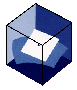BOOKSCurriculum Vitae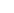Books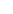Books in EnglishEdited booksBooks in DanishAncestorsWMY 2000RPAMathMathematicsBooks in EnglishEdited booksBooks in Danish   Fundamental Concepts in Modern Analysis: An Introduction to Nonlinear Analysis (2nd ed.)Many applied mathematical disciplines, such as dynamical systems and optimization theory as well as classical mathematical disciplines like differential geometry and the theory of Lie groups, have a common foundation in general topology and multivariate calculus in normed vector spaces. In this book, students from both pure and applied subjects are offered an opportunity to work seriously with fundamental notions from mathematical analysis that are important not only from a mathematical point of view but also occur frequently in the theoretical parts of, for example, the engineering sciences. The book provides complete proofs of the basic results from topology and differentiability of mappings in normed vector spaces. It is a useful resource for students and researchers in mathematics and the many sciences that depend on fundamental techniques from mathematical analysis. In this second edition, the notions of compactness and sequentially compactness are developed with independent proofs for the main results. Thereby the material on compactness is apt for direct applications also in functional analysis, where the notion of sequentially compactness prevails. This edition also covers a new section on partial derivatives, and new material has been incorporated to make a more complete account of higher order derivatives in Banach spaces, including full proofs for symmetry of higher order derivatives and Taylor's formula. The exercise material has been reorganized from a collection of problem sets at the end of the book to a section at the end of each chapter with further results. Readers will find numerous new exercises at different levels of difficulty for practice. Read more     Functional Analysis: Entering Hilbert Space (2nd ed.)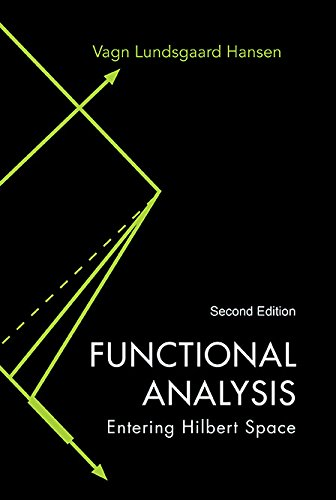This book presents basic elements of the theory of Hilbert spaces and operators on Hilbert spaces, culminating in a proof of the spectral theorem for compact, self-adjoint operators on separable Hilbert spaces. It exhibits a construction of the space of pth power Lebesgue integrable functions by a completion procedure with respect to a suitable norm in a space of continuous functions, including proofs of the basic inequalities of Hölder and Minkowski. The Lp-spaces thereby emerges in direct analogy with a construction of the real numbers from the rational numbers. This allows grasping the main ideas more rapidly. Other important Banach spaces arising from function spaces and sequence spaces are also treated. In this second edition, I have expanded the material on normed vector spaces and their operators presented in Chapter 1 to include proofs of the Open Mapping Theorem, the Closed Graph Theorem and the Hahn–Banach Theorem. The material on operators between normed vector spaces is further expanded in a new Chapter 6, which presents the basic elements of the theory of Fredholm operators on general Banach spaces, not only on Hilbert spaces. This requires that we develop the theory of dual operators between Banach spaces to replace the use of adjoint operators between Hilbert spaces. With the addition of the new material on normed vector spaces and their operators, the book can serve as a general introduction to functional analysis viewed as a theory of infinite dimensional linear spaces and linear operators acting on them. Read more     Functional Analysis: Entering Hilbert Space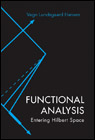This book presents basic elements of the theory of Hilbert spaces and operators on Hilbert spaces, culminating in a proof of the spectral theorem for compact, self-adjoint operators on separable Hilbert spaces. It exhibits a construction of the space of pth power Lebesgue integrable functions by a completion procedure with respect to a suitable norm in a space of continuous functions, including proofs of the basic inequalities of Hölder and Minkowski. The Lp-spaces thereby emerges in direct analogy with a construction of the real numbers from the rational numbers. This allows grasping the main ideas more rapidly. Other important Banach spaces arising from function spaces and sequence spaces are also treated. Read more     Matematikkens Uendelige UniversDenne bog henvender sig til alle der ønsker et indblik i matematikkens mange bidrag til kultur, videnskab og samfund. Bogen er derfor tilrettelagt som en række små essays, hvor der tilstræbes en mere beskrivende og fortællende fremstilling end normalt i bøger med et matematisk indhold. Emnerne i bogen er mangfoldige og strækker sig fra beskrivelse af geometriske former og fænomener i omverdenen til diskussion af nogle af uendelighedsbegrebets mange aspekter. Store dele af bogen kræver ikke specielle matematiske forkundskaber, men nok en vis optagethed af faget. Bogen giver i glimt smagsprøver på matematikkens abstrakte idčverden, herunder et indblik i nogle af matematikkens nyeste landvindinger.     Fundamental Concepts in Modern AnalysisMany advanced mathematical disciplines, such as dynamical systems, calculus of variations, differential geometry and the theory of Lie groups, have a common foundation in general topology and calculus in normed vector spaces. In this book, mathematically inclined engineering students are offered an opportunity to go into some depth with fundamental notions from mathematical analysis that are not only important from a mathematical point of view but also occur frequently in the more theoretical parts of the engineering sciences. The book should also appeal to university students in mathematics and in the physical sciences. Read more